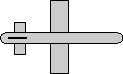# Maths - Conversion Axis-Angle to Euler

## Prerequisites

Definition of terms:

## Equations

heading = atan((x * y * (1 - cos(angle)) + z * sin(angle)) / (1 - (y2 + z2 ) * (1 - cos (angle)))
bank = atan((y * z * (1 - cos(angle)) + x * sin(angle)) / (1 - (x2 + y2)* (1 - cos (angle)))
attitude = asin(- (x * z * (1 - cos(angle)) - y * sin(angle))

x

## Derivation of Equations

Euler angles represent 3 rotations about the x,y and z axis in some given order. We can replace any sequence of rotations by one single rotation about some axis.

We need to be very careful about using Euler Angles and it is best to work in terms of quaternions or matricies whenever we can. As explained in euler section there are different types of Euler angles and the result will depend on the Euler definition used. Also the following calculations use a lot of trig functions and therefor will use a lot of CPU time. The accuracy may be low, specially near the singularity of the Euler system being used.

With these warnings in mind here is the calculation.

Euler can be defined in terms of a quaternion as shown here.

heading = atan(2.0 * (qx*qy + qz*qw)/(qx2 - qy2 - qz2 + qw2))
bank = atan(2.0 * (qy*qz + qx*qw)/(-qx2 - qy2 + qz2 + qw2))
attitude = asin(-2.0 * (qx*qz - qy*qw))

This quaternion can be defined in terms of axis-angle as shown here.

qx = x * sin(angle/2);
qy = y * sin(angle/2);
qz = z * sin(angle/2);
qw = cos(angle/2)

So working out some products gives:

qx*qw = x * sin(angle/2) * cos(angle/2) = 0.5 * x * sin(angle) // using trig identity: sin(angle) = 2 sin(angle/2) cos(angle/2) from here

qy*qw = y * sin(angle/2) * cos(angle/2) = 0.5 * y * sin(angle)

qz*qw = z * sin(angle/2) * cos(angle/2) = 0.5 * z * sin(angle)

qx*qy = x * y * sin2(angle/2) = x * y * (0.5 - 0.5*cos(angle)) // using trig identity cos(angle) = 1 - 2 sin2(angle/2) from here

qy*qz = y * z * sin2(angle/2) = y * z * (0.5 - 0.5*cos(angle))

qx*qz = x * z * sin2(angle/2) = x * z * (0.5 - 0.5*cos(angle))

qx2 = x2 * sin2(angle/2) = x2 * (0.5 - 0.5*cos(angle))

qy2 = y2 * sin2(angle/2) = y2 * (0.5 - 0.5*cos(angle))

qz2 = z2 * sin2(angle/2) = z2 * (0.5 - 0.5*cos(angle))

qw2 =cos2(angle/2) = 0.5 * (cos(angle)+1) // using trig identity cos(2A) = 2 cos2(A) - 1 from here

qx2 - qy2 - qz2 + qw2
= qx2 + qy2 + qz2 + qw2 - 2qy2 - 2qz2
= 1 - 2qy2 - 2qz2
= 1 - y2 * (1 - cos(angle)) - z2 * (1 - cos(angle))
= 1 - (y2 + z2 ) * (1 - cos (angle))

-qx2 - qy2 + qz2 + qw2
= qx2 + qy2 + qz2 + qw2 -2qx2 - 2qy2
= 1 - 2qx2 - 2qy2
= 1 - x2 * (1 - cos (angle)) - y2 * (1 - cos (angle))
= 1 - (x2 + y2)* (1 - cos (angle))

So substituting in euler equations gives:

heading = atan(2.0 * (qx*qy + qz*qw)/(qx2 - qy2 - qz2 + qw2))
bank = atan(2.0 * (qy*qz + qx*qw)/(-qx2 - qy2 + qz2 + qw2))
attitude = asin(-2.0 * (qx*qz - qy*qw))

heading = atan(2.0 * (x * y * (0.5 - 0.5*cos(angle)) + 0.5 * z * sin(angle)) / (1 - (y2 + z2 ) * (1 - cos (angle)))
bank = atan(2.0 * (y * z * (0.5 - 0.5*cos(angle)) + 0.5 * x * sin(angle)) / (1 - (x2 + y2)* (1 - cos (angle)))
attitude = asin(-2.0 * (x * z * (0.5 - 0.5*cos(angle)) - 0.5 * y * sin(angle))

heading = atan((x * y * (1 - cos(angle)) + z * sin(angle)) / (1 - (y2 + z2 ) * (1 - cos (angle)))
bank = atan((y * z * (1 - cos(angle)) + x * sin(angle)) / (1 - (x2 + y2)* (1 - cos (angle)))
attitude = asin(- (x * z * (1 - cos(angle)) - y * sin(angle))

## Example

 we take the 90 degree rotation from this:to this:As shown here the axis angle for this rotation is:

angle = 90 degrees
axis = 1,0,0

So using the above result:

heading = atan((x * y * (1 - cos(angle)) + z * sin(angle)) / (1 - (y2 + z2 ) * (1 - cos (angle)))
bank = atan((y * z * (1 - cos(angle)) + x * sin(angle)) / (1 - (x2 + y2)* (1 - cos (angle)))
attitude = asin(- (x * z * (1 - cos(angle)) - y * sin(angle))

we substitute our axis angle: angle=90 degrees, x=1, y=0, z=0

heading = atan(0 / 1 )
bank = atan(1 / 0)
attitude = asin(0 )

which gives: